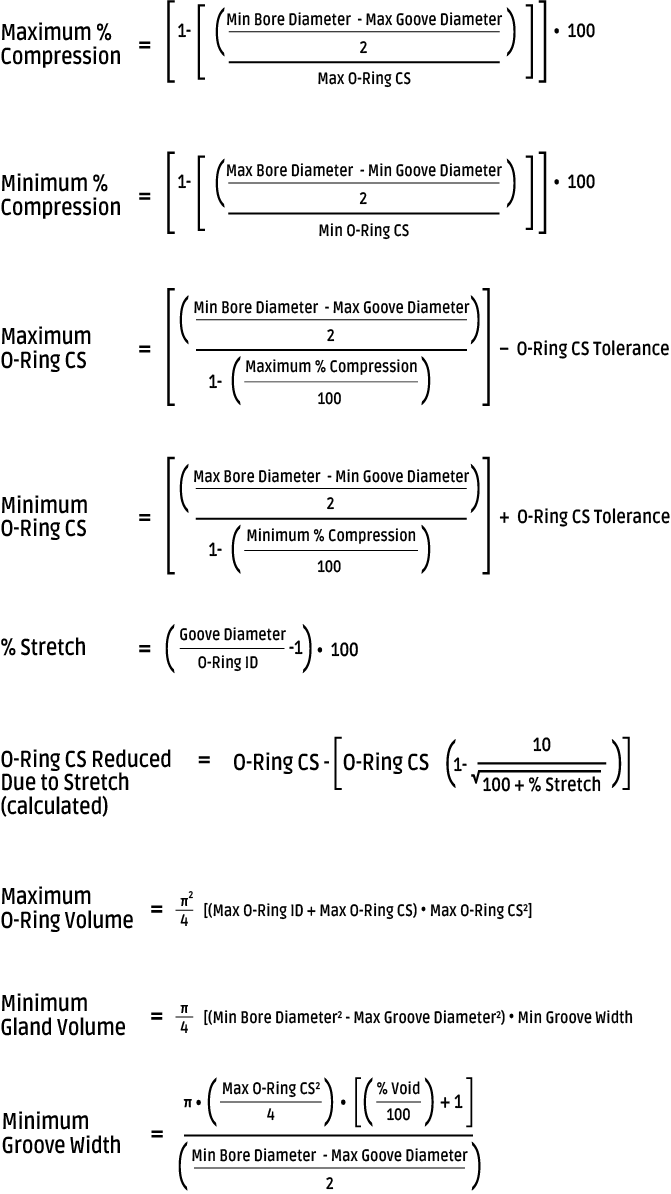*NOTE: The value "% Void" is typically a minimum of 10%.

## Conversion Table

Length Temperature 1 mm = 0.039 in.1 m = 3.281 ft. 1 in = 25.4 mm1 ft = 0.305 m Temp (°C) = 0.56 (°F -32) Temp (°F) = 1.8 °C +32 1 Pa = 1 N / m2 = 1.4504 x 10-4 psi 1 bar = 105 N / m2 = 14.5 psi 1 atm = 1.01325 bar = 14.696 psi 1 psi = 6894.8 Pa

*NOTE: All formulas assume concentricity and most apply to a Piston configuration.